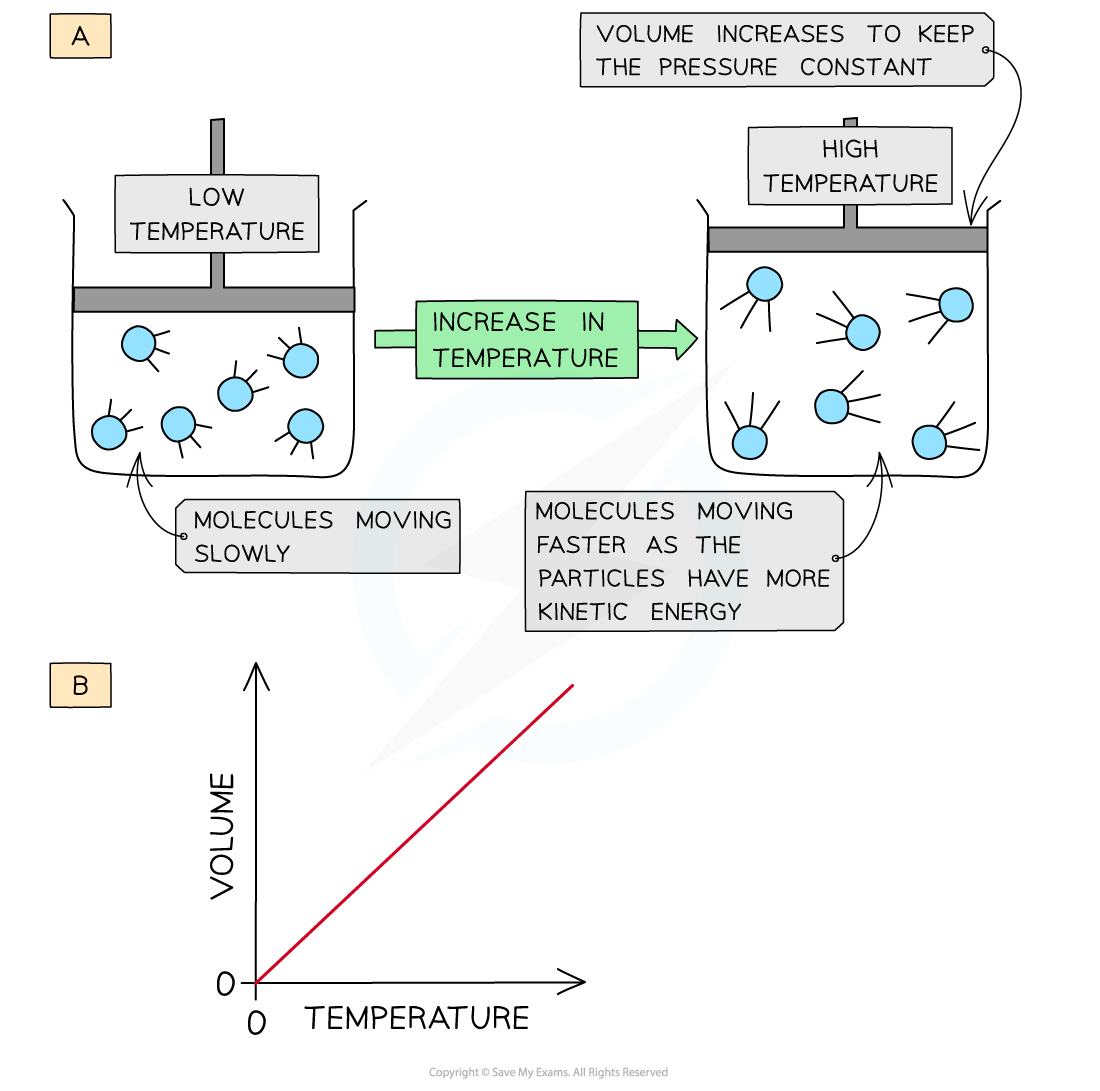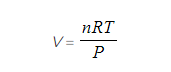# AQA A Level Chemistry复习笔记1.3.4 The Ideal Gas Equation

### The Ideal Gas Equation

#### Kinetic theory of gases

• The kinetic theory of gases states that molecules in gases are constantly moving
• The theory makes the following assumptions:
• That gas molecules are moving very fast and randomly
• That molecules hardly have any volume
• That gas molecules do not attract or repel each other (no intermolecular forces)
• No kinetic energy is lost when the gas molecules collide with each other (elastic collisions)
• The temperature of the gas is related to the average kinetic energy of the molecules

• Gases that follow the kinetic theory of gases are called ideal gases
• However, in reality gases do not fit this description exactly but may come very close and are called real gases

#### Ideal gases

• The volume that an ideal gas occupies depends on:
• Its pressure
• Its temperature

• When a gas is heated (at constant pressure) the particles gain more kinetic energy and undergo more frequent collisions with the container wall
• To keep the pressure constant, the molecules must get further apart and therefore the volume increases
• The volume is therefore directly proportional to the temperature (at constant pressure)The volume of a gas increases upon heating to keep a constant pressure (a); volume is directly proportional to the temperature (b)

#### Limitations of the ideal gas law

• At very low temperatures and high pressures real gases do not obey the kinetic theory as under these conditions:
• Molecules are close to each other
• There are instantaneous dipole- induced dipole or permanent dipole- permanent dipole forces between the molecules
• These attractive forces pull the molecules away from the container wall
• The volume of the molecules is not negligible

• Real gases therefore do not obey the following kinetic theory assumptions at low temperatures and high pressures:
• There is zero attraction between molecules (due to attractive forces, the pressure is lower than expected for an ideal gas)
• The volume of the gas molecules can be ignored (volume of the gas is smaller than expected for an ideal gas)

#### Ideal gas equation

• The ideal gas equation shows the relationship between pressure, volume, temperature and number of moles of gas of an ideal gas:

#### PV = nRT

P = pressure (pascals, Pa)

V = volume (m3)

n = number of moles of gas (mol)

R = gas constant (8.31 J K-1 mol-1)

T = temperature (kelvin, K)

#### Worked Example

Calculating the volume of a gas

Calculate the volume occupied by 0.781 mol of oxygen at a pressure of 220 kPa and a temperature of 21 °C

Step 1: Rearrange the ideal gas equation to find volume of gasStep 2: Calculate the volume the oxygen gas occupies

• P = 220 kPa = 220 000 Pa
• n = 0.781 mol
• R = 8.31 J K-1 mol-1
• T = 21 oC = 294 K#### Worked Example

Calculating the molar mass of a gas

A flask of volume 1000 cm3 contains 6.39 g of a gas. The pressure in the flask was 300 kPa and the temperature was 23 °C.

Calculate the relative molecular mass of the gas.

Step 1: Rearrange the ideal gas equation to find the number of moles of gasStep 2: Calculate the number of moles of gas

p = 300 kPa = 300 000 Pa

V = 1000 cm3 = 1 dm3 = 0.001 m3

R = 8.31 J K-1 mol-1

T = 23 oC = 296 KStep 3: Calculate the molar mass using the number of moles of gas#### Exam Tip

To calculate the temperature in Kelvin, add 273 to the Celsius temperature - e.g. 100 oC is 373 Kelvin.

You must be able to rearrange the ideal gas equation to work out all parts of it.

The units are incredibly important in this equation - make sure you know what units you should use, and do the necessary conversions when doing your calculations!